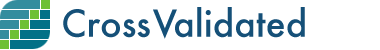R versus Python tags - quotient Py/R

0

(click on this box to dismiss)Q&A for statisticians, data analysts, data miners and data visualization experts

``````select
cast(cast(t.yr as varchar(4))+'-'+cast(t.mnth as varchar(2))+'-01' as date) as dt
,cast(t.py_mnth_count as float)/cast(t.r_mnth_count as float) as py_div_r
from
(
select
month(CreationDate) as mnth
,year(CreationDate) as yr
-- count <r> tags
,sum(case when tags like '%<r>%' then 1 else 0 end) as r_mnth_count
-- count <python> tags
,sum(case when tags like '%<python>%' then 1 else 0 end) as py_mnth_count
from
posts
where
-- tags are wrapped by <>
tags like '%<r>%'
or tags like '%<python>%'
group by month(CreationDate), year(CreationDate)
) as t
-- exclude last (incomplete) month
where not (t.mnth=month(GETDATE()) and t.yr=year(GETDATE()))
and yr>=2011
order by t.yr asc, t.mnth asc``````

Enter Parameters

Options:
-Hold tight while we fetch your results
:records returned in :time ms:cached